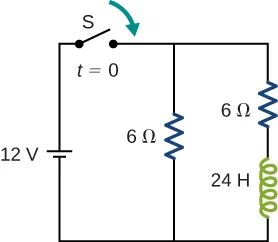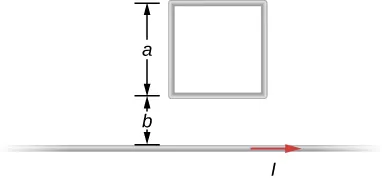University Physics Volume 2

# Challenge Problems

University Physics Volume 2Challenge Problems

### Challenge Problems

82.

A coaxial cable has an inner conductor of radius a, and outer thin cylindrical shell of radius b. A current I flows in the inner conductor and returns in the outer conductor. The self-inductance of the structure will depend on how the current in the inner cylinder tends to be distributed. Investigate the following two extreme cases. (a) Let current in the inner conductor be distributed only on the surface and find the self-inductance. (b) Let current in the inner cylinder be distributed uniformly over its cross-section and find the self-inductance. Compare with your results in (a).

83.

In a damped oscillating circuit the energy is dissipated in the resistor. The Q-factor is a measure of the persistence of the oscillator against the dissipative loss. (a) Prove that for a lightly damped circuit the energy, U, in the circuit decreases according to the following equation.

$dUdt=−2βU,dUdt=−2βU,$ where $β=R2L.β=R2L.$

(b) Using the definition of the Q-factor as energy divided by the loss over the next cycle, prove that Q-factor of a lightly damped oscillator as defined in this problem is 

$Q ≡ U begin Δ U one cycle = 1 2 π R L C . Q ≡ U begin Δ U one cycle = 1 2 π R L C .$

(Hint: For (b), to obtain Q, divide E at the beginning of one cycle by the change $ΔEΔE$ over the next cycle.)

84.

The switch in the circuit shown below is closed at $t=0st=0s$. Find currents through (a) $R1R1$, (b) $R2R2$, and (c) the battery as function of time.85.

A square loop of side 2 cm is placed 1 cm from a long wire carrying a current that varies with time at a constant rate of 3 A/s as shown below. (a) Use Ampère’s law and find the magnetic field. (b) Determine the magnetic flux through the loop. (c) If the loop has a resistance of $3Ω3Ω$, how much induced current flows in the loop?Order a print copy

As an Amazon Associate we earn from qualifying purchases.